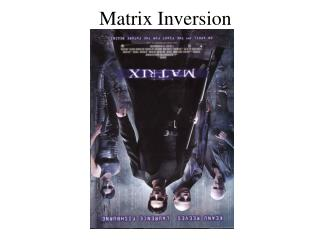DownloadDownload PresentationMatrix Inversion

# Matrix Inversion

Télécharger la présentation## Matrix Inversion

- - - - - - - - - - - - - - - - - - - - - - - - - - - E N D - - - - - - - - - - - - - - - - - - - - - - - - - - -
##### Presentation Transcript

1. Matrix Inversion

2. Definition • The inverse of an n by n matrix A is an n by n matrix B where, AB = BA = In. • Please note: Not all matrices have inverses! Singular matrices don’t have inverse. • If a matrix has an inverse, then it is called invertible.

3. Definition If A is a square matrix, and if a matrix B of the same size can be found such that AB=BA=I , then A is said to be invertible and B is called an inverse of A . If no such matrix B can be found, then A is said to be singular . Notation:

4. Properties of Matrix Inverse • If A is an invertible matrix then its inverse is unique. • (A-1)-1 = A. • (Ak)-1= (A-1)k (we will denote this as A-k ) • (cA)-1 = (1/c)A-1, c ≠ 0. • ( AT)-1 = (A-1)T. • If A is an invertible matrix, then the system of equations Ax = b has a unique solution given by x = A-1b.

5. The Transpose of a Matrix • A is the m×n matrix given by • The transpose matrix of A, denoted by AT, is a n×m matrix given by

6. The inverse of a 2-by-2 matrix • For a 2-by-2 matrix,

7. The inverse of a 2 x 2 matrix • From this we deduced that a 2x2 matrix A is singular if and only if ad-bc = 0. • This quantity (ad-bc) has some other useful properties as well and so is defined to be the determinant of the matrix A.

8. Determinants of larger matrices • There is no “nice” formula for the inverse of larger than 2x2 matrices. • We still can define the determinantof a larger square matrix and it will still have the property that the determinant of A= 0 if and only if A is singular. • First we need some terminology.

9. Minors and cofactors • If A is a square matrix, then the minorMijof the element aijof A is the determinant of the matrix obtained by deleting the i-th row and the j-th column from A. • The cofactorCij = (-1)i+jMij.

10. Definition of a Determinant If A is a square matrix of order 2 or greater, then the determinant of A is the sum of the entries in the first row of A multiplied by their cofactors. That is,

11. Determinant of 2-by-2 Matrix

12. Determinant of 3-by-3 Matrix

13. Matrix Inversion How to calculate the matrix inverse? What is an adjoint matrix?

14. Adjoint Matrix – Minors and Cofactors • The adjoint matrix of [A], Adj[A] is obtained by taking the transpose of the cofactor matrix of [A]. • The minor for element aijof matrix [A] is found by removing the ith row and jth column from [A] and then calculating the determinant of the remaining matrix.

15. Matrix Inversion Consider the following set of linear equations. The coefficients can be arranged in a matrix form as shown.

16. Matrix Inversion The set of equations in matrix form is:

17. Minors

18. Minors The resulting matrix of minors is:

19. Cofactors Cofactors are the signed minors. The cofactor of element aijof matrix [A] is: Therefore The resulting matrix of cofactors is:

20. Adjoint matrix The adjoint matrix of [A], Adj[A] is obtained by taking the transpose of the cofactor matrix of [A]. Evaluate the determinant

21. Matrix Inversion

22. Matrix Inversion Using G-J Elimination If Gauss–Jordan elimination is applied on a square matrix, it can be used to calculate the matrix's inverse. This can be done by augmenting the square matrix with the identity matrix of the same dimensions., and through the following matrix operations:

23. If the original square matrix, A, is given by the following expression: Then, after augmenting by the identity, the following is obtained:

24. By performing elementary row operations on the [AI] matrix until A reaches reduced row echelon form, the following is the final result: The matrix augmentation can now be undone, which gives the following: